# Forced Vibration of Single Degree of Freedom Systems (Part - 2) Civil Engineering (CE) Notes | EduRev

## Civil Engineering (CE) : Forced Vibration of Single Degree of Freedom Systems (Part - 2) Civil Engineering (CE) Notes | EduRev

The document Forced Vibration of Single Degree of Freedom Systems (Part - 2) Civil Engineering (CE) Notes | EduRev is a part of the Civil Engineering (CE) Course Introduction to Dynamics and Vibrations- Notes, Videos, MCQs.
All you need of Civil Engineering (CE) at this link: Civil Engineering (CE)

5.4.4 Features of the Steady State Response of Spring Mass Systems to Forced Vibrations

Now, we will discuss the implications of the results in the preceding section.

• The steady state response is always harmonic, and has the same frequency as that of the forcing.

To see this mathematically, note that in each case the solution has the form x(t) = X0 sin(ωt+φ). Recall that ω defines the frequency of the force, the frequency of base excitation, or the rotor angular velocity. Thus, the frequency of vibration is determined by the forcing, not by the properties of the spring-mass system. This is unlike the free vibration response.

You can also check this out using our applet. To switch off the transient solution, click on the checkbox labeled `show transient’. Then, try running the applet with different values for k, m and λ , as well as different forcing frequencies, to see what happens. As long as you have switched off the transient solution, the response will always be harmonic.

• The amplitude of vibration is strongly dependent on the frequency of excitation, and on the properties of the spring—mass system.

To see this mathematically, note that the solution has the form x(t) = X0 sin(ωt+φ). Observe that X0 is the amplitude of vibration, and look at the preceding section to find out how the amplitude of vibration varies with frequency, the natural frequency of the system, the damping factor, and the amplitude of the forcing. The formulae for X0 are quite complicated, but you will learn a great deal if you are able to sketch graphs of X0 as a function of ω / ωn for various values of ζ .

You can also use our applet to study the influence of forcing frequency, the natural frequency of the system, and the damping coefficient. If you plot position-v-time curves, make sure you switch off the transient solution to show clearly the steady state behavior. Note also that if you click on the `amplitude –v- frequency’ radio button just below the graphs, you will see a graph showing the steady state amplitude of vibration as a function of forcing frequency. The current frequency of excitation is marked as a square dot on the curve (if you don’t see the square dot, it means the frequency of excitation is too high to fit on the scale – if you lower the excitation frequency and press `start’ again you should see the dot appear). You can change the properties of the spring mass system (or the natural frequency and damping coefficient) and draw new amplitude-v-frequency curves to see how the response of the system has changed.

Try the following tests

(i) Keeping the natural frequency fixed (or k and m fixed), plot ampltude-v-frequency graphs for various values of damping coefficient (or the dashpot coefficient). What happens to the maximum amplitude of vibration as damping is reduced?

(ii) Keep the damping coefficient fixed at around 0.1. Plot graphs of amplitude-v-frequency for various values of the natural frequency of the system. How does the maximum vibration amplitude change as natural frequency is varied? What about the frequency at which the maximum occurs?

(iii) Keep the dashpot coefficient fixed at a lowish value. Plot graphs of amplitude-v-frequency for various values of spring stiffness and mass. Can you reconcile the behavior you observe with the results of test (ii)?

(iv) Try changing the type of forcing to base excitation and rotor excitation. Can you see any differences in the amplitude-v-frequency curves for different types of forcing?

(v) Set the damping coefficient to a low value (below 0.1). Keep the natural frequency fixed. Run the program for different excitation frequencies. Watch what the system is doing. Observe the behavior when the excitation frequency coincides with the natural frequency of the system. Try this test for each type of excitation.

• If the forcing frequency is close to the natural frequency of the system, and the system is lightly damped, huge vibration amplitudes may occur. This phenomenon is known as resonance.

If you ran the tests in the preceding section, you will have seen the system resonate. Note that the system resonates at a very similar frequency for each type of forcing.

As a general rule, engineers try to avoid resonance like the plague. Resonance is bad vibrations, man. Large amplitude vibrations imply large forces; and large forces cause material failure. There are exceptions to this rule, of course. Musical instruments, for example, are supposed to resonate, so as to amplify sound. Musicians who play string, wind and brass instruments spend years training their lips or bowing arm to excite just the right vibration modes in their instruments to make them sound perfect.

• There is a phase lag between the forcing and the system response, which depends on the frequency of excitation and the properties of the spring-mass system.

The response of the system is x(t) = X0 sin(ωt+φ) . Expressions for φ are given in the preceding section. Note that the phase lag is always negative.

You can use the applet to examine the physical significance of the phase lag. Note that you can have the program plot a graph of phase-v-frequency for you, if you wish.

It is rather unusual to be particularly interested in the phase of the vibration, so we will not discuss it in detail here.

5.4.5 Engineering implications of vibration behavior

The solutions listed in the preceding sections give us general guidelines for engineering a system to avoid (or create!) vibrations.

Preventing a system from vibrating: Suppose that we need to stop a structure or component from vibrating – e.g. to stop a tall building from swaying. Structures are always deformable to some extent – this is represented qualitatively by the spring in a spring-mass system. They always have mass – this is represented by the mass of the block. Finally, the damper represents energy dissipation. Forces acting on a system generally fluctuate with time. They probably aren’t perfectly harmonic, but they usually do have a fairly well defined frequency (visualize waves on the ocean, for example, or wind gusts. Many vibrations are man-made, in which case their frequency is known – for example vehicles traveling on a road tend to induce vibrations with a frequency of about 2Hz, corresponding to the bounce of the car on its suspension).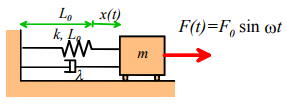So how do we stop the system from vibrating? We know that its motion is given by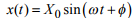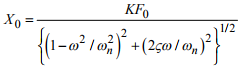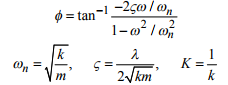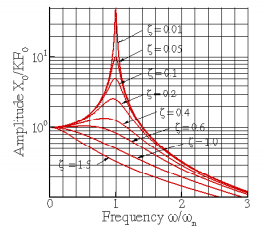To minimize vibrations, we must design the system to make the vibration amplitude X0 as small as possible. The formula for X0 is a bit scary, which is why we plot graphs of the solution. The graphs show that we will observe vibrations with large amplitudes if (i) The frequency ω / ωn is close to 1; and (ii) the damping ζ is small. At first sight, it looks like we could minimize vibrations by making ω / ωn very large. This is true in principle, and can be done in some designs, e.g. if the force acts on a very localized area of the structure, and will only excite a single vibration mode. For most systems, this approach will not work, however. This is because real components generally have a very large number of natural frequencies of vibration, corresponding to different vibration modes. We could design the system so that ω / ωn is large for the mode with the lowest frequency – and perhaps some others – but there will always be other modes with higher frequencies, which will have smaller values of ω / ωn . There is a risk that one of these will be close to resonance. Consequently, we generally design the system so that ω / ωn <<1 for the mode with the lowest natural frequency. In fact, design codes usually specify the minimum allowable value of ω / ωn for vibration critical components. This will guarantee that ω / ωn <<1 for all modes, and hence the vibration amplitude X0 → KF0 = F0 / K . This tells us that the best approach to avoid vibrations is to make the structure as stiff as possible. This will make the natural frequency large, and will also make F0 / k small.

Designing a suspension or vibration isolation system. Suspensions, and vibration isolation systems, are examples of base excited systems. In this case, the system really consists of a mass (the vehicle, or the isolation table) on a spring (the shock absorber or vibration isolation pad). We expect that the base will vibrate with some characteristic frequency ω. Our goal is to design the system to minimize the vibration of the mass.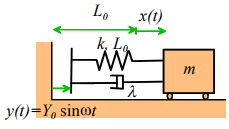Our vibration solution predicts that the mass vibrates with displacement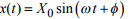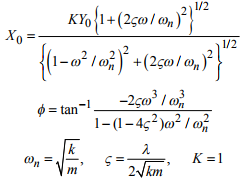Again, the graph is helpful to understand how the vibration amplitude X0 varies with system parameters.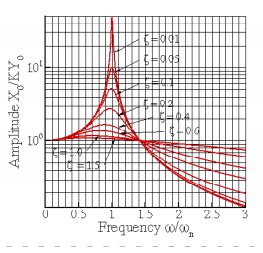Clearly, we can minimize the vibration amplitude of the mass by making ω / ωn <<1. We can do this by making the spring stiffness as small as possible (use a soft spring), and making the mass large. It also helps to make the damping ζ small. This is counter-intuitive – people often think that the energy dissipated by the shock absorbers in their suspensions that makes them work. There are some disadvantages to making the damping too small, however. For one thing, if the system is lightly damped, and is disturbed somehow, the subsequent transient vibrations will take a very long time to die out. In addition, there is always a risk that the frequency of base excitation is lower than we expect – if the system is lightly damped, a potentially damaging resonance may occur.

Suspension design involves a bit more than simply minimizing the vibration of the mass, of course – the car will handle poorly if the wheels begin to leave the ground. A very soft suspension generally has poor handling, so the engineers must trade off handling against vibration isolation.

5.4.6 Using Forced Vibration Response to Measure Properties of a System.

We often measure the natural frequency and damping coefficient for a mode of vibration in a structure or component, by measuring the forced vibration response of the system.

Here is how this is done. We find some way to apply a harmonic excitation to the system (base excitation might work; or you can apply a force using some kind of actuator, or you could deliberately mount an unbalanced rotor on the system).

Then, we mount accelerometers on our system, and use them to measure the displacement of the structure, at the point where it is being excited, as a function of frequency.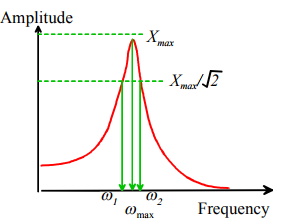We then plot a graph, which usually looks something like the picture on the right. We read off the maximum response Xmax , and draw a horizontal line at amplitude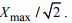Finally, we measure the frequencies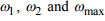as shown in the picture.

We define the bandwidth of the response Δω as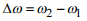Like the logarithmic decrement, the bandwidth of the forced harmonic response is a measure of the damping in a system.

It turns out that we can estimate the natural frequency of the system and its damping coefficient using the following formulae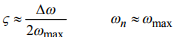The formulae are accurate for small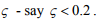To understand the origin of these formulae, recall that the amplitude of vibration due to external forcing is given by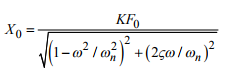We can find the frequency at which the amplitude is a maximum by differentiating with respect to ω , setting the derivative equal to zero and solving the resulting equation for frequency. It turns out that the maximum amplitude occurs at a frequency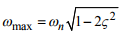For small ζ , we see tha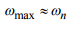Next, to get an expression relating the bandwidth Δω to ζ, we first calculate the frequencies ω1 and ω2 Note that the maximum amplitude of vibration can be calculated by setting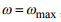, which gives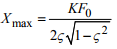Now, at the two frequencies of interest, we know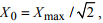so that ω1 and ω2 must be solutions of the equation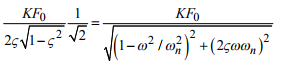Rearrange this equation to see that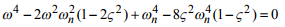This is a quadratic equation for ω2 and has solutions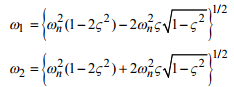Expand both expressions in a Taylor series about ζ = 0 to see that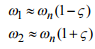so, finally, we confirm that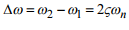5.4.7 Example Problems in Forced Vibrations

Example 1: A structure is idealized as a damped spring—mass system with stiffness 10 kN/m; mass 2Mg; and dashpot coefficient 2 kNs/m. It is subjected to a harmonic force of amplitude 500N at frequency 0.5Hz. Calculate the steady state amplitude of vibration.

Start by calculating the properties of the system: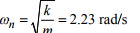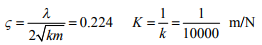Now, the list of solutions to forced vibration problems gives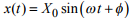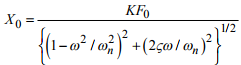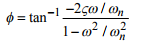For the present problem: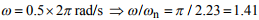Substituting numbers into the expression for the vibration amplitude shows that

X0 = 43 mm

Example 2: A car and its suspension system are idealized as a damped spring—mass system, with natural frequency 0.5Hz and damping coefficient 0.2. Suppose the car drives at speed V over a road with sinusoidal roughness. Assume the roughness wavelength is 10m, and its amplitude is 20cm. At what speed does the maximum amplitude of vibration occur, and what is the corresponding vibration amplitude?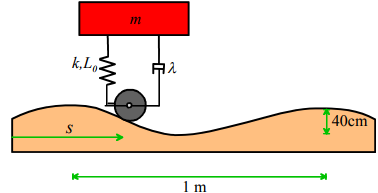Let s denote the distance traveled by the car, and let L denote the wavelength of the roughness and H the roughness amplitude. Then, the height of the wheel above the mean road height may be expressed as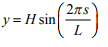Noting that s = Vt , we have that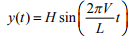i.e., the wheel oscillates vertically with harmonic motion, at frequency ω = 2πV / L.

Now, the suspension has been idealized as a spring—mass system subjected to base excitation. The steady state vibration is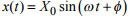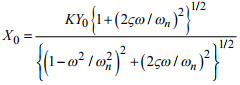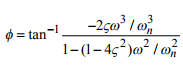For light damping, the maximum amplitude of vibration occurs at around the natural frequency. Therefore, the critical speed follows from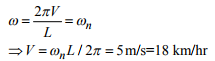Note that K=1 for base excitation, so that the amplitude of vibration at ω / ωn = 1 is approximately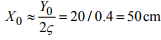Note that at this speed, the suspension system is making the vibration worse. The amplitude of the car’s vibration is greater than the roughness of the road. Suspensions work best if they are excited at frequencies well above their resonant frequencies.

Example 3: The suspension system discussed in the preceding problem has the following specifications. For the roadway described in the preceding section, the amplitude of vibration may not exceed 35cm at any speed. At 55 miles per hour, the amplitude of vibration must be less than 10cm. The car weighs 3000lb. Select values for the spring stiffness and the dashpot coefficient.

We must first determine values for ζ and ωn that will satisfy the design specifications. To this end:

(i) The specification requires that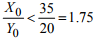at resonance. Examine the graph of X0 / KY0 shown with the solutions to the equations of motion. Recall that K=1 for a base excited spring—mass system. Observe that, with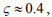the amplification factor never exceeds 1.75.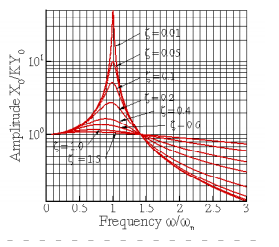(ii) Now, the frequency of excitation at 55mph is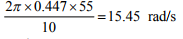We must choose system parameters so that, at this excitation frequency, X0 / Y0 < 10 / 20 = 1 / 2. Examine the graph showing the response of a base excited spring—mass system again. We observe that, for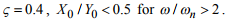Therefore, we pick ωn = ω / 2 = 7.7 rad/s.

Finally, we can compute properties of the system. We have that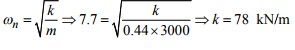Similarly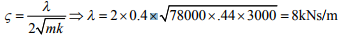Offer running on EduRev: Apply code STAYHOME200 to get INR 200 off on our premium plan EduRev Infinity!

## Introduction to Dynamics and Vibrations- Notes, Videos, MCQs

20 videos|53 docs

,

,

,

,

,

,

,

,

,

,

,

,

,

,

,

,

,

,

,

,

,

;